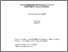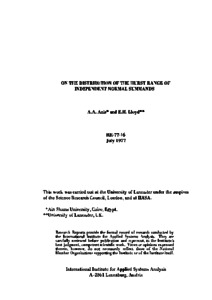On the Distribution of the Hurst Range of Independent Normal Summands

Anis, A.A. & Lloyd, E.H. (1977). On the Distribution of the Hurst Range of Independent Normal Summands. IIASA Research Report. IIASA, Laxenburg, Austria: RR-77-016Preview
Text
RR-77-016.pdf

Abstract

The well-known empirical findings of H.E. Hurst have inspired a vast quantity of research on the ability of various mathematical models of geophysical time series to reproduce the Hurst effect. Anis and Lloyd have earlier obtained an exact result for the expected value of the Hurst range when the inflow process is a sequence of independent and identically distributed normal variables. The research described in the present report is an attempt to extend this so as to obtain an explicit formula for the probability distribution of the Hurst range for this process.

A general method is developed, and explicit results are obtained for the cases n less than or equal to 4 (n denoting the number of summands considered). These distributions are of an unusual form, the probability density functions showing discontinuities and corners. Graphs are provided showing the quantitative behavior of these functions.

It is surmised that the problem of deriving exact explicit formulae for the distribution of Hurst's range for general values of n is of unmanageable complexity.

Item Type: Monograph (IIASA Research Report) Resources and Environment Area (REN) IIASA Import 15 Jan 2016 01:44 27 Aug 2021 17:08 http://pure.iiasa.ac.at/699View Item